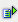The function abs (num) returns as a result the absolute value of a number. The absolute value of a number is the value without sign.

Syntax: =abs(num)

num: is the real number which is the basis for the absolute valueExample =abs(2.2) is 2 =abs(-2.2) is 2